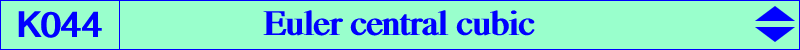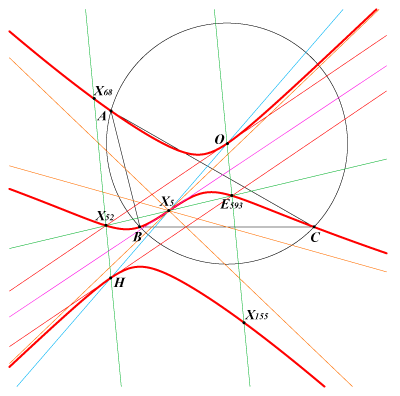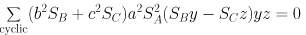X(3), X(4), X(5), X(52), X(68), X(155), X(5562) feet of altitudes and their reflections in X(5) (these points on the perpendicular bisectors) reflections of A, B, C in X(5) : centers of circles HBC, HCA, HAB i.e. centers of the Johnson circles infinite points of pK(X6, X5889) points on the circumcircle and on pK(X6, X14516) their reflections in X(5), on C(H, R)The Euler cubic is the central pK with center X(5), pivot X(4), pole X(216). It is the Darboux cubic of the orthic triangle. See the related K627. It is the locus of point M such that the parallels at M to AO, BO, CO meet the corresponding sidelines of ABC in three points forming a triangle perspective to ABC. The locus of the perspector is K045, a remarkable isotomic pK with pivot X(264) called the Euler perspector cubic. See other locus properties in the page K646 and here (Angel Montesdeoca), also K612 and K674 (César Lozada), and Table 76. The isogonal transform of K044 is K919.